# Selina Concise Maths Solution for ICSE Class 10 Chapter 25 Probability

## Selina Class 10 Maths solutions for chapter 25

This chapter consists of a variety of topics of probability in which problems are expected in exams. These concepts are given in a very comprehensive manner in ICSE Solutions For Class 10 Maths. Probability is the measure of uncertainty of various phenomenon, numerically. It can have a positive value from 0 to 1. The words ‘probably’, ‘doubt’, ‘most probably’, ‘chances’, etc., used in the statements above involve an element of uncertainty. Single Event: If an event E has only one sample point of a sample space, it is called a simple (or elementary) event. In a sample space containing n distinct elements, there are exactly n simple events. E.g. in rolling a die, Simple event could be the event of getting 4

Check out the Class 10 Selina Maths ICSE Solutions Chapter 25 Probability which is given below: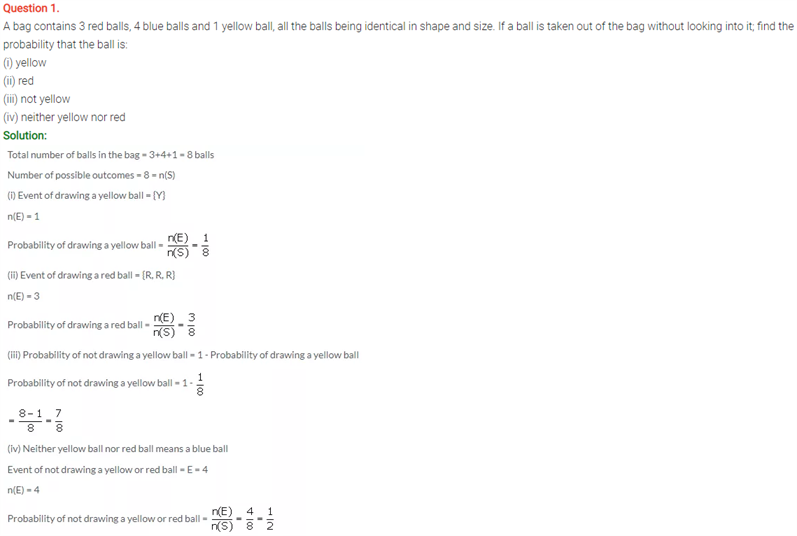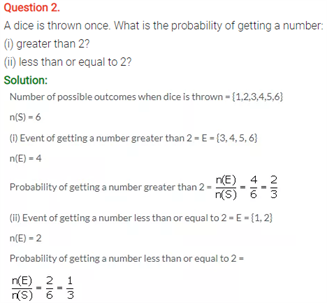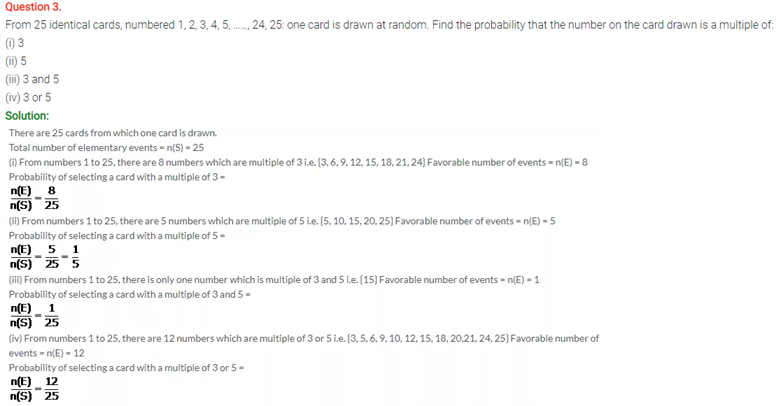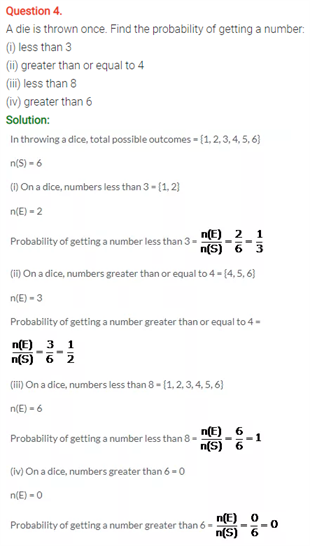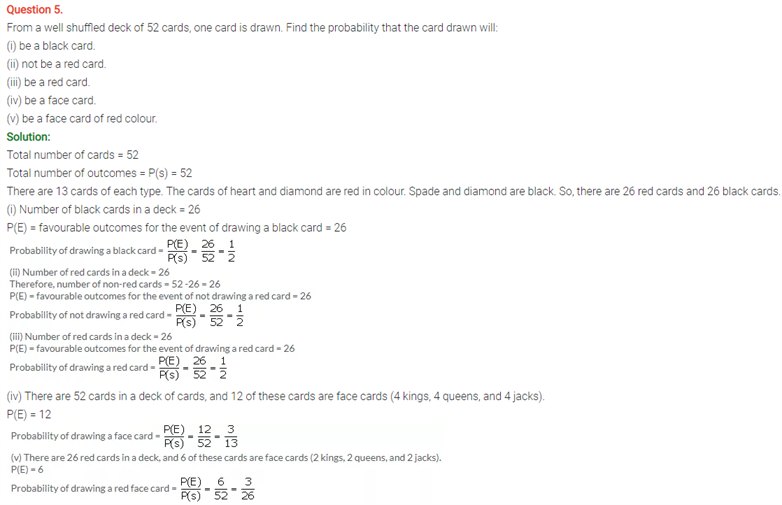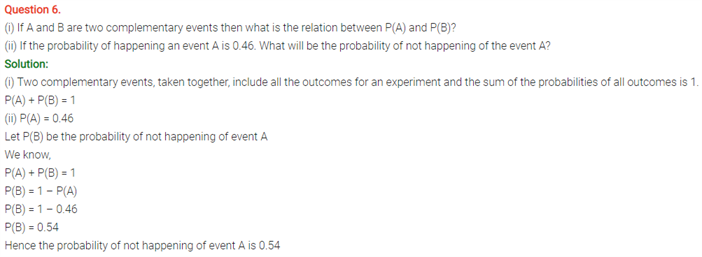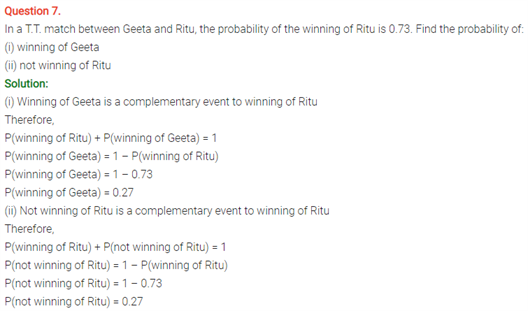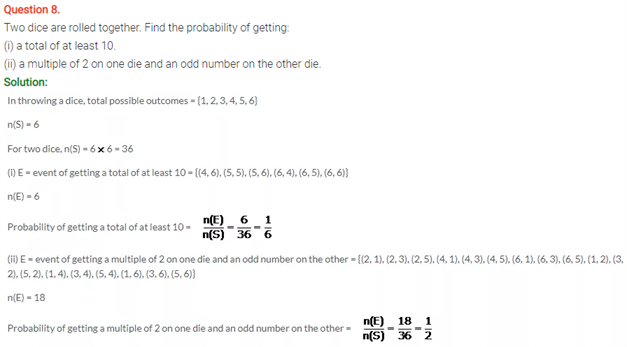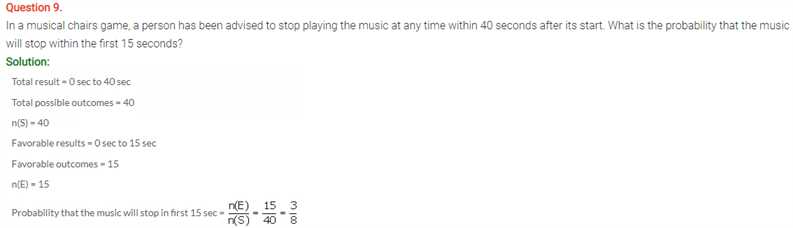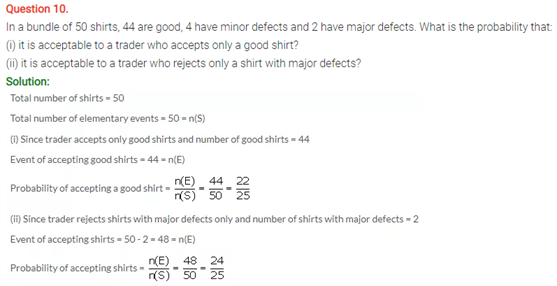1. Leeza khan

Good app